• The data are standardized by subtracting group means and dividing by pooled standard deviations.
 • The singular value decomposition is used to obtain a principal component transformation matrix from the set of singular vectors.
 • The number of components retained represents a minimum of 0.9999 of the sum of the squared singular values.
 • A linear discriminant analysis is performed on the transformed data, where the data are not shifted by group means. This is a fast calculation because the pooled-within covariance matrix is diagonal.
This section gives the derivation of formulas saved by Score Options > Save Formulas. The formulas depend on the Discriminant Method.
For each group defined by the categorical variable X, observations on the covariates are assumed to have a p-dimensional multivariate normal distribution, where p is the number of covariates. The notation used in the formulas is given in Notation for Formulas Given by Save Formulas Options.
 p number of covariates T total number of groups (levels of X) t = 1, ..., T subscript to distinguish groups defined by X nt n = n1 + n2 + ... + nT total number of observations y p by 1 vector of covariates for an observation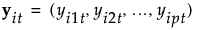ith observation in group t, consisting of a vector of p covariates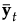p by 1 vector of means of the covariates y for observations in group t ybar p by 1 vector of means for the covariates across all observations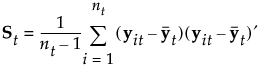estimated (p by p) within-group covariance matrix for group t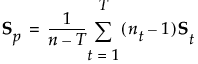estimated (p by p) pooled within covariance matrix qt p(t|y) posterior probability that y belongs to group t |A| determinant of a matrix A
The Mahalanobis distance from an observation y to group t is defined as follows:
The likelihood for an observation y in group t is estimated as follows:
Note that the number of parameters that must be estimated is p2 for the pooled covariance matrix plus pT for the means.
An observation y is assigned to the group for which its posterior probability is the largest.
 SqDist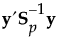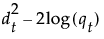Prob[]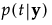Pred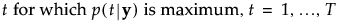In quadratic discriminant analysis, the within-group covariance matrices are not assumed equal. The within-group covariance matrix for group t is estimated by St. This means that the total number of parameters to be estimated is Tp2 + Tp: Tp2 for the within-group covariance matrices and Tp for the means.
When group sample sizes are small relative to p, the estimates of the within-group covariance matrices tend to be highly variable. The discriminant score is heavily influenced by the smallest eigenvalues of the inverse of the within-group covariance matrices. See Friedman, 1989. For this reason, if your group sample sizes are small compared to p, you might want to consider the Regularized method, described in Regularized Discriminant Method.
See Notation for Formulas Given by Save Formulas Options for notation. The Mahalanobis distance from an observation y to group t is defined as follows:
The likelihood for an observation y in group t is estimated as follows:
An observation y is assigned to the group for which its posterior probability is the largest.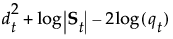Prob[]PredNote: SqDist[<group t>] can be negative.
 • The parameter λ balances weights assigned to the pooled covariance matrix and the within-group covariance matrices, which are not assumed equal.
 • The parameter γ determines the amount of shrinkage toward a diagonal matrix.
The Mahalanobis distance from an observation y to group t is defined as follows:
The likelihood for an observation y in group t is estimated as follows:
An observation y is assigned to the group for which its posterior probability is the largest.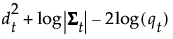Prob[]PredNote: SqDist[<group t>] can be negative.
The Wide Linear method is useful when you have a large number of covariates and, in particular, when the number of covariates exceeds the number of observations (p > n). This approach centers around an efficient calculation of the inverse of the pooled within-covariance matrix Sp or of its transpose, if p > n. It uses a singular value decomposition approach to avoid inverting and allocating space for large covariance matrices.
See Notation for Formulas Given by Save Formulas Options for notation. The steps in the Wide Linear calculation are as follows:
 1 Compute the T by p matrix M of within-group sample means. The (t,j)th entry of M, mtj, is the sample mean for members of group t on the jth covariate.
 2 For each covariate j, calculate the pooled standard deviation across groups. Call this sjj.
 3
 4 Center and scale values for each covariate as follows:
 ‒ Subtract the mean for the group to which the observation belongs.
 ‒ Divide the difference by the pooled standard deviation.
Using notation, for an observation i in group t, the group-centered and scaled value for the jth covariate is:
The notation t(i) indicates the group t to which observation i belongs.
 5
 6 Denote the pooled within-covariance matrix for the group-centered and scaled covariates by R. The matrix R is given by the following:
 7
where U and V are orthonormal and D is a diagonal matrix with positive entries (the singular values) on the diagonal. See The Singular Value Decomposition in Statistical Details.
Then R can be written as follows:
 8 If R is of full rank, obtain R-1/2 as follows:
where D-1 is the diagonal matrix whose diagonal entries are the inverses of the diagonal entries of D.
If R is not of full rank, define a pseudo-inverse for R as follows:
 9 If R is of full rank, it follows that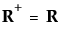. So, for completeness, the discussion continues using pseudo-inverses.
Define a p by p matrix Ts as follows:
The formulas for the Mahalanobis distance, the likelihood, and the posterior probabilities are identical to those in Linear Discriminant Method. However, the inverse of Sp is replaced by a generalized inverse computed using the singular value decomposition.
When you save the formulas, the Mahalanobis distance is given in terms of the decomposition. For an observation y, the distance to group t is the following, where the last equality uses the notation seen in the saved formulas:
 Discrim Data Matrix Vector of observations on the covariates Discrim Prin Comp SqDist Sum of squares of the entries in the matrix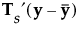Prob[] Pred